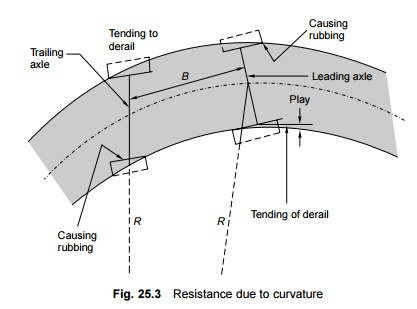Home | | Railways and Airports and Harbour Engineering | Train Resistance Due to Curvature

# Train Resistance Due to CurvatureWhen a train negotiates a horizontal curve, extra effort is required to overcome the resistance offered by the curvature of the track. Curve resistance is caused basically because of the following reasons.

Various forces offer resistance to the movement of a train on the track. These resistances may be a result of the movement of the various parts of the locomotives as well as the friction between them, the irregularities in the track profile, or the atmospheric resistance to a train moving at great speed. The tractive power of a locomotive should be adequate enough to overcome these resistances and haul the train at a specified speed.

Resistance Due to Curvature

When a train negotiates a horizontal curve, extra effort is required to overcome the resistance offered by the curvature of the track. Curve resistance is caused basically because of the following reasons (Fig. 25.3).

The vehicle cannot adapt itself to a curved track because of its rigid wheel base. This is why the frame takes up a tangential position as vehicle tries to move in a longitudinal direction along the curve as shown in Fig. 25.3. On account of this, the flange of the outer wheel of the leading axle rubs against the inner face of the outer rail, giving rise to resistance to the movement of the train.(b) Curve resistance can sometimes be the result of longitudinal slip, which causes the forward motion of the wheels on a curved track. The outer wheel flange of the trailing axle remains clear and tends to derail. The position worsens further if the wheel base is long and the curve is sharp.

(c)  Curve resistance is caused when a transverse slip occurs, which increases the friction between the wheel flanges and the rails.

(d) Poor track maintenance, particularly bad alignment, worn out rails, and improper levels, also increase resistance.

(e)  Inadequate superelevation increases the pressure on the outer rail and, similarly, excess superelevation puts greater pressure on the inner rails, and this also contributes to an increase in resistance.

The value of curve resistance can be determined by the following equation:

Curve resistance = C . FG/R

where F is the force of sliding friction, G is the gauge of the track, R is the mean radius of the curve, and C is the constant, which is dependent on various factors. This equation indicates that

(a)  curve resistance increases with increase in gauge width and

(b) resistance is inversely proportional to the radius, i.e., it increases with an

increase in the degree of the curve.

Empirical formulae have been worked out for curve resistance, which are as follows:

Curve resistance for BG (R5) = 0.0004WD         (25.7)

Curve resistance for MG (R5) = 0.0003WD        (25.8)

Curve resistance for NG (R5) = 0.0002WD         (25.9)

where W is the weight of the train in tonnes and D is the degree of the curve. It means that for a 4 o curve on a BG line, the curve resistance for a train weighing 250 t would be 0.0004 � 250 � 4 = 0.4 t.

Curve resistance is quite often compensated or offset by a reduction in the gradient. In this way, the effect of curve resistance is translated in terms of resistance due to gradient. The compensation is 0.04% on BG, 0.03% on MG, and 0.02% on NG lines for every 1 o of the curve. This will be clear through the solved example given below.

Example 25.1 Calculate the compensated gradient on a BG track with a 4 o curvature on a ruling gradient of 1 in 200.

Solution

Ruling gradient = 1 in 200 = 0.5% Compensation for curvature = 0.04 � 4 = 0.16%

Compensated gradient = 0.05 - 0.16 = 0.34% = 1 in 294

Special characteristics of curve resistance

Certain characteristics of curve resistance have been observed over the years. These are enumerated here.

(a)  Curve resistance increases with the speed of the train.

(b) Curve resistance depends upon the central angle of the curve. For example, if there are two curves of different radii, but with the same central angle, the total curve resistance would be the same.

(c)  Though curve resistance depends on the weight of the train, the length of the train has no appreciable effect on it.

(d) Curve resistance is less with new rails compared to that with old rails.

Depending upon various factors, curve resistance can be as low as 0.20 kg per tonne per degree curve when the track is in good condition and may go as high as 1.0 kg per tonne per degree curve for worn out rails, rough tracks, and other unfavourable conditions of the track.

Study Material, Lecturing Notes, Assignment, Reference, Wiki description explanation, brief detail
Civil : Railway Airport Harbour Engineering : Railway Engineering : Train Resistance and Tractive Power : Train Resistance Due to Curvature |

Related Topics